# Oversimplified: Signals and Systems (3) – Prereq: Linear Algebra

‘Signals and Systems’ (the title of Oppenheim and Wilsky’s book) class is also called ‘Linear Signals and Systems’ (by B.P. Lathi). The keyword of the day is ‘linear‘. It’s the same ‘linear’ as in linear algebra!

Most ideas in this class are very basic linear algebra ideas rolled out in its most tedious, explicit long form because they assumed you haven’t taken linear algebra yet*. I think it’s a horrible compromise because:

• there’s too much information to organize in your head and remember. Not compact!
• it’s a huge intellectual jump when you get to modern (as in state-space) approach later.
• with computer data, you end up using linear algebra implicitly in this class anyway.

By emphasizing the concept of linearity at the beginning, you can take advantage of the linear algebra tools (especially inner products) to simplify the ideas in this class to a few things that you can remember and interpret in seconds.

Before I start, I’d like to emphasize that ‘signals’ and ‘systems’ are the same mathematical object (vector or function) so the math is interchangeable. Nonetheless it’s often useful to call the inputs/outputs ‘signals’ and the function map ‘systems’ to give so insights to the context of the problem at hand. In this class, ‘systems’ are almost always linear, so it’s a linear function map.

Most definitions and usages IN THIS CLASS allows you to interchange the roles ‘signals’ and ‘systems’ as the math is symmetric (swapping them will give you the same results = commutative).

Most frequently, a signal/system is most often described as a function of time, but it doesn’t have to be, as any arbitrary (scalar or vector) inputs with any meaning (such as distance) will do. If only spotty time instances are recorded, we call it a discrete-time signal, or time-series.

The word ‘linear’ actually has a strict mathematical definition. Out of infinite possible functions (systems or map)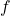, if we declare it to be linear, we are limiting it to the ones satisfying ALL of the following: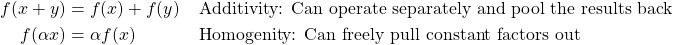Verify by yourself the following commonly used linear operators are indeed linear by the definitions above. Try making up examples on your own:

• Derivative (e.g.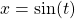,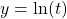)
• Integral
• Expectation (aka the mean)

These imposed restrictions makes the math much much easier, something an engineer so eagerly desires that they will try to bend the problem to make the system almost linear. It goes under the name of linearization. Transistors amplifies with approximate linearity.

Also note that an affine function, which is a linear function plus a constant offset, is NOT linear. Linear functions has to cross the origin. An example would be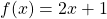. Try the definition above with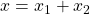, you will get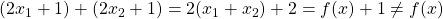. The constant offset is added twice instead of once!

Students often think the definition of linearity is stating the obvious, and didn’t pay too much attention to it. Turns out it’s one of the golden tricks for the class called superposition. You can use these properties freely in this class because almost everything (except a few trick problems) is declared linear.

As with superposition in LINEAR circuits, you can shut-off any combinations of individual ADDITIVE input components (sources), process them separately with the same system, then pool (add) the individual outputs together as the overall output.

ONLY with linear systems, the outputs caused by different input components (however way you want to break it) will have nothing to do with each other (no coupling) as far as the output is concerned. If they couple, you are looking at a non-linear system, which is nasty to work with as you have to watch out for all possible interactions.

A useful alternative way of saying the same thing is that any signal can be seen as a linear combination of multiple sources (feel free to define the sources/basis that are convenient for you). I kept it generic here because you will see the same concept in convolution as well as all the transforms in this class.

Now go and grab a basic linear algebra book and learn these basics, really understanding them:

• Elementary matrices (identity matrices, elementary vectors)
• Vector spaces (what I just covered above: superposition! Understand basis well)
• Linear transformations (they’re 100% of what linear systems are. Know inverses)
• Orthorgonality and INNER PRODUCTS (it’s the compact form the transforms in this class)

These can wait until you take the linear algebra class**:

• Gaussian elimination (goes under row echelon form), determinants
• Similarity transformations, eigen-decomposition / SVD
• Quadratic forms and least squares
• Gram-Schmidt (QR) factorization and numerical linear algebra.

I recommend the 4th or 5th edition of Steve Leon’s “Linear Algebra with Applications” because it’s short and gets to the point very quickly. Anything more is just clutter.

The only important idea that’s missing from Leon’s book, which is covered in the first lecture by Gilbert Strang is this:

• Left multiplication by a matrix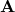over input vector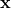, i.e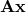means scaling each column of,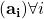by the weights (elements) of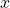and add them all up: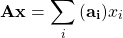This is the foundation of ‘basis’, which I’ll explain later how it relates to all the transformations in this class.

* Gilbert Strang propose that the math curriculum can simply start with linear algebra without Calculus. The neat thing about them is they don’t depend on each other, so you can learn it in different order.

So far almost all schools starts with calculus because it was historically way more established than linear algebra. Integrals and derivatives are linear operators, which can be expressed in matrices as well, so I can say linear algebra are no way less powerful. Nowadays, since most digital information is stored in vectors, they are more readily consumed by tools of linear algebra than calculus, so I expect the balance of power will change in the coming decades.

What the gateway ‘signals and systems’ class teaches is the ‘classic’ approach, which is heavy on the 4 basic transforms written in calculus forms. The ‘modern’ approach, which is always taught in state-space control, sees everything as matrices and vectors: each of the 4 transforms are nothing but left multiplying by a matrix (a linear map). As we will see later, DFT (Discrete Fourier Transform) is just left multiplying your data (vector) by a matrix.

** These are very important materials if you get deep into signal processing, but won’t help you in the gateway class. For example, the connection between eigenvalues and transformations in the class is a very advanced topic way after this class. Least squares are crucial when you get to statistical signal processing.## 3 thoughts on “Oversimplified: Signals and Systems (3) – Prereq: Linear Algebra”

1.Jez on said:

Your “Oversimplified Series” helps me look at Signal Processing in a very different light. It’s like you have mentioned, I hated the subject. But I am trying to re-learn it again through your series. Looking forward to more. Thank you so much. I am very grateful. Keep it up.Report

2.admin on said:

Thanks. I’ll write more about it!

Hope this ‘series’ will help you to see the subject in an intuitive and easy way, *after* you built the minimal background in linear algebra (this post) and the complex numbers properties (the previous one).

Make sure you understand inner products first. It’s under the hood of all the transforms in typical signals-and-systems classes (they are all linear transforms).

Give signal processing another shot! It’s not a hard subject: it’s just so badly structured that nobody learned it right the first time.Report

3.Jez on said:

Yes, definitely! (on building the background and giving it another shot)
Thanks again.Report